## 1. 引言

“一对一”的逻辑关系是指：

• 对于其中的结点，有且仅有一个开始结点没有前驱&但有一个后继结点
• 有且仅有一个终端结点没有后继&但有一个前驱节点
• 其余所有的结点都有且仅有一个前驱和后继

## 2. 队列和栈

### 2.1 原理和特点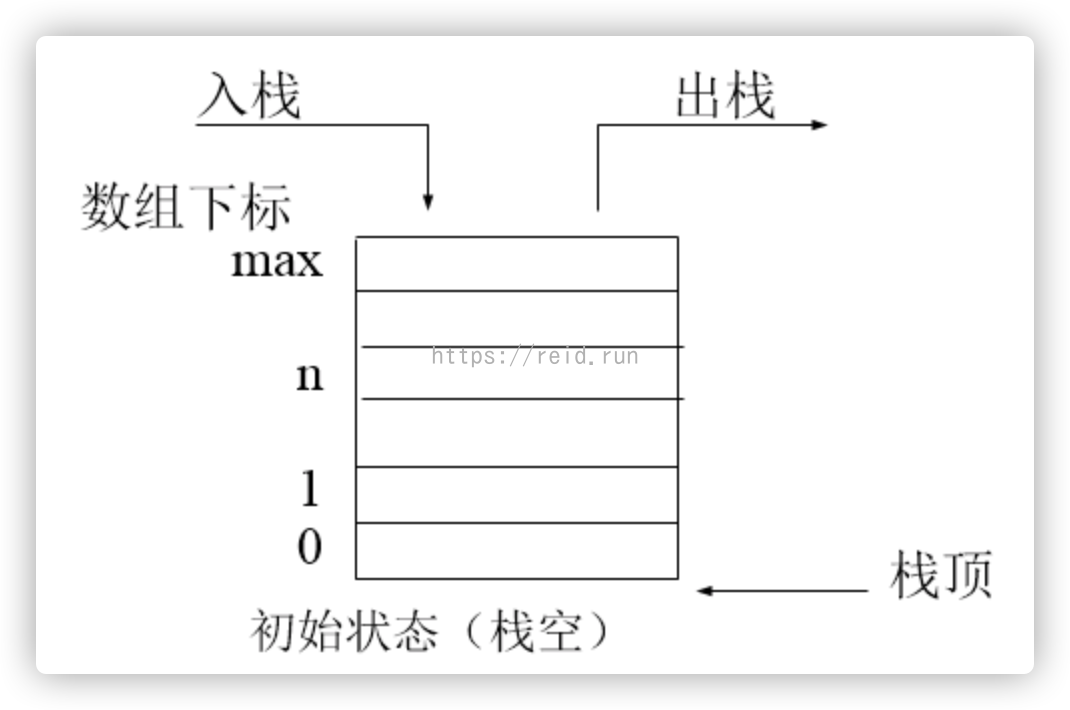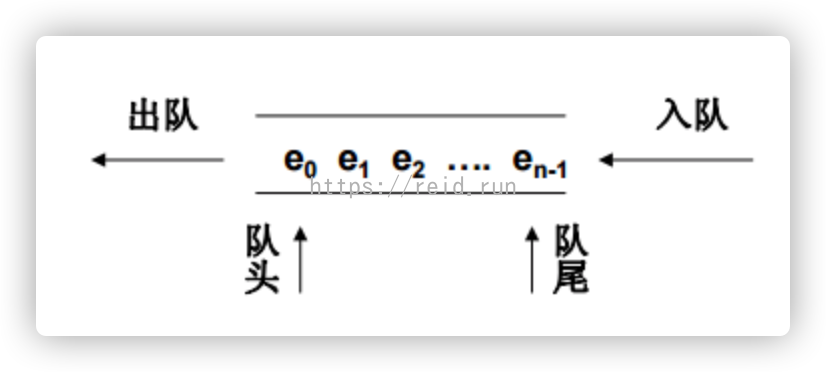• 栈的插入和删除操作只允许在尾端进行，栈中称为：栈顶，也满足FIFO(First in Last Out)
• 队列的只允许在队尾插入数据，在队头删除数据，也满足FIFO(First in Last Out)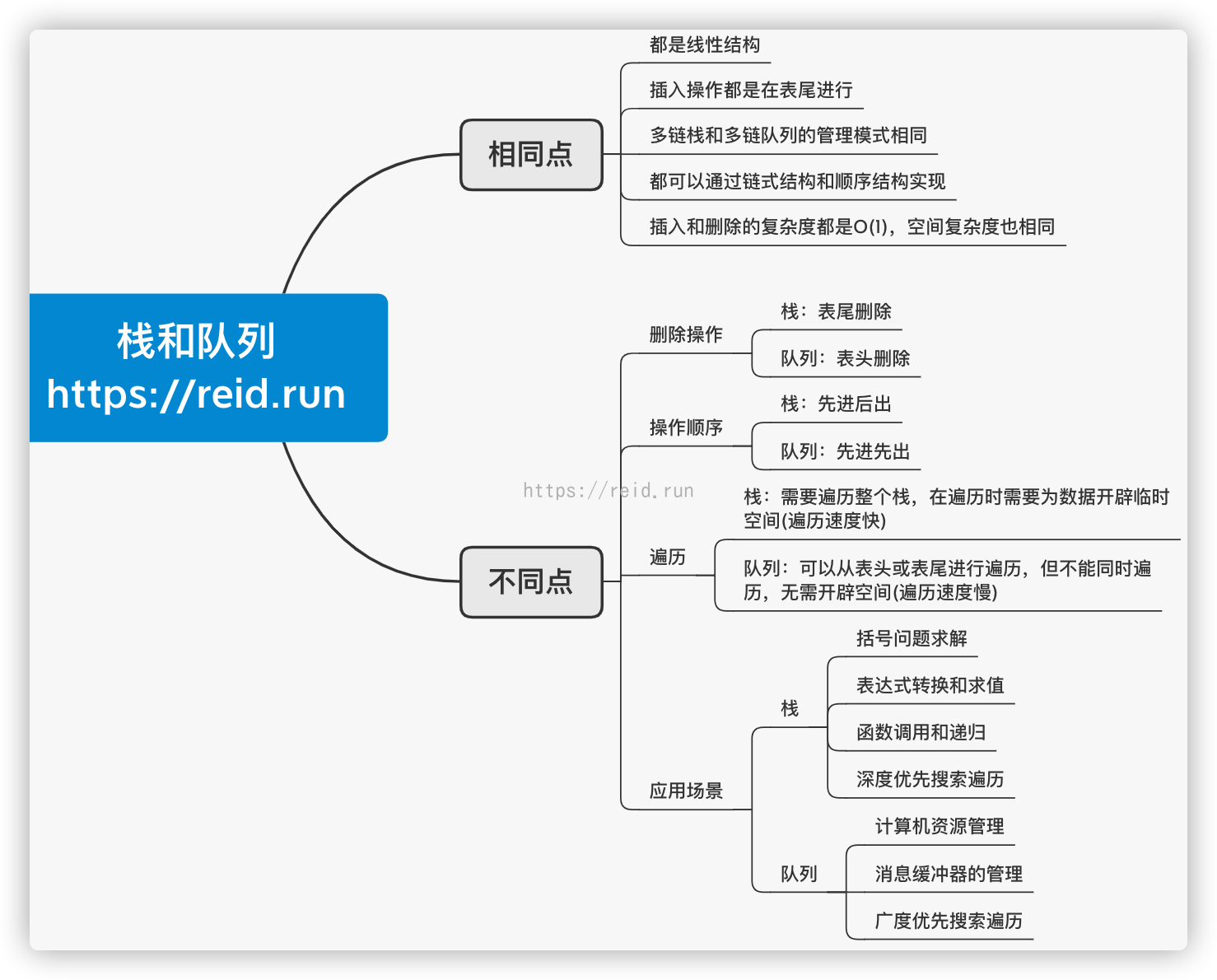### 2.2 实现

#### 2.2.1 栈的实现一：列表

List列表是Python中的基础数据结构，我们可以使用List的append()pop()方法来模拟入栈、出栈操作。

stack = []  # 模拟一个栈
# 入栈
stack.append(2)
print(stack)
stack.append(4)
print(stack)
stack.append(6)
print(stack)
# 查看栈顶元素
top = stack[-1]
print('栈顶元素为：{}'.format(top))
# 出栈
for i in range(len(stack)):
t = stack.pop()
print('出栈元素为：',t)
if not stack:
print('此时栈为：[空]')
else:
print('此时栈中还有 {} 个元素'.format(len(stack)))
# 输出

[2, 4]
[2, 4, 6]



#### 2.2.2 栈的实现二：双向队列

Python中的内置模块collections中包含了双向队列deque的数据类型，可以在队列的左端和右端实现入队和出队。

from collections import deque
stack = deque()  # 模拟一个栈
# 入栈
stack.append(2)
print(stack)
stack.append(4)
print(stack)
stack.append(6)
print(stack)
# 查看栈顶元素
top = stack[-1]
print('栈顶元素为：{}'.format(top))
# 出栈
print('栈中元素为：',list(stack))
for i in range(len(stack)):
t = stack.pop()
print('出栈元素为：',t)
if not stack:
print('此时栈为：[空]')
else:
print('此时栈中还有 {} 个元素'.format(len(stack)))
# 输出
deque()
deque([2, 4])
deque([2, 4, 6])



#### 2.2.3 队列的实现一：列表

stack = []  # 模拟一个栈
# 入栈
stack.append(2)
print(stack)
stack.append(4)
print(stack)
stack.append(6)
print(stack)
# 查看栈顶元素
top = stack[-1]
print('栈顶元素为：{}'.format(top))
# 出栈
for i in range(len(stack)):
t = stack.pop(0)
print('出栈元素为：',t)
if not stack:
print('此时栈为：[空]')
else:
print('此时栈中还有 {} 个元素'.format(len(stack)))
# 输出

[2, 4]
[2, 4, 6]



#### 2.2.4 栈的实现二：deque

from collections import deque
stack = deque()  # 模拟一个栈
# 入栈
stack.append(2)
print(stack)
stack.append(4)
print(stack)
stack.append(6)
print(stack)
# 查看栈顶元素
top = stack[-1]
print('栈顶元素为：{}'.format(top))
# 出栈
print('栈中元素为：',list(stack))
for i in range(len(stack)):
t = stack.popleft()  # 没有popright()方法
print('出栈元素为：',t)
if not stack:
print('此时栈为：[空]')
else:
print('此时栈中还有 {} 个元素'.format(len(stack)))
# 输出
deque()
deque([2, 4])
deque([2, 4, 6])



## 3. 堆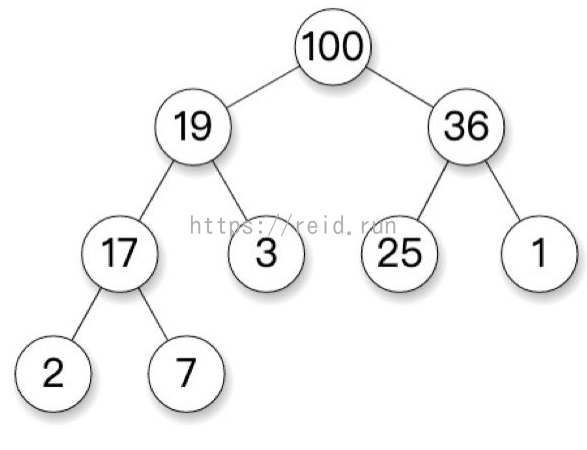### 3.1 实现

Python中内置了堆的模块heapq直接导入就能够调用。

import heapq

# 列表q
q = [100,19,36,7,3,25,1,2,17]
# 对q进行最大堆排序
heapq._heapify_max(q)
print('最大堆排序为:',q) # 看看是不是和图片结果相同呢？
# 对q进行最小堆排序
heapq.heapify(q)
print('最小堆排序为:',q)
# 删除堆顶元素
a = heapq.heappop(q)
print('堆顶元素为:',a)
print('堆排序为:',q)
# 在堆中加入一个元素num，并对堆进行重排序
num = 1
heapq.heappush(q,num)
print('堆排序为:',q)

#在堆中加入一个元素，保持堆元素数量不变，如果加入的元素大于堆顶元素则删除堆顶元素
nums = 4
heapq.heapreplace(q,nums)
print('堆排序为:',q)
# 输出



## 4. 吐槽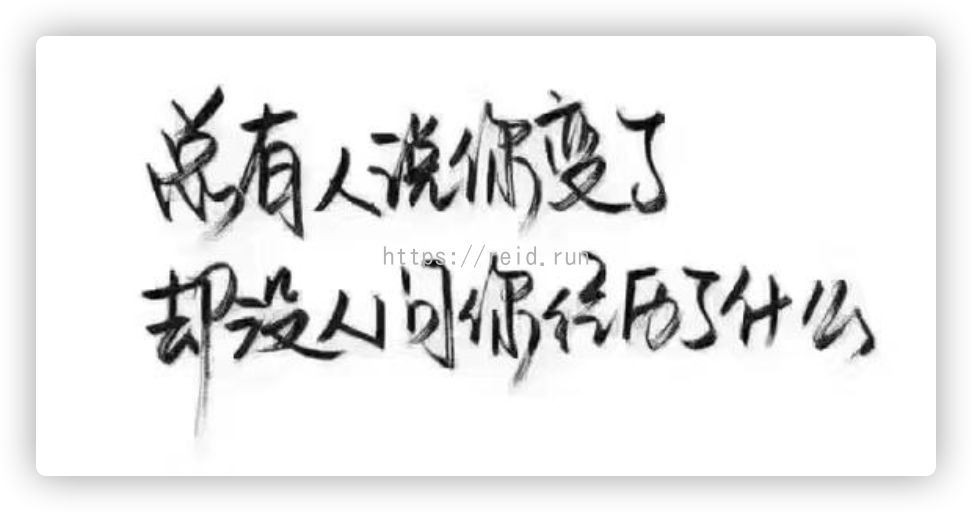Q.E.D.

print("种一棵树最好的时间是十年前，其次是现在")